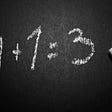# Angling for Vacation with Spherical Geometry

## Gems in STEM: The Basics of Spherical Geometry (Pt. 2)

Last time, I left you all on a bit of a cliff-hanger (totally my bad). In particular, we had defined the basic tools of spherical geometry (i.e. great circles), but didn’t answer some other questions about other objects on spheres! For example, if there are no straight lines in this geometry, what happens to angles? Or shapes? (Note: If you’re not sure what spherical geometry is about, I recommend you first read my last article which introduces everything we work with here!)

First, a warning: FLAT-EARTHERS BEWARE, THE FOLLOWING (SCIENTIFICALLY ACCURATE) INFORMATION MAY BE UPSETTING.

Now, let’s start with the first question: angles. In traditional (Euclidean) geometry, an angle is defined by the space between two intersecting straight lines. In spherical geometry, the concept of straight lines is replaced by great circles. So, as expected, an angle between two lines in spherical geometry is defined by the corresponding great circles; specifically, it is the angle between the planes of the great circles. For example, the angle between the two great circles in the below diagram is θ.

Now that we know what angles are, we are able to tackle something a little more difficult: spherical trigonometry, an area of spherical geometry that is concerned with how trig functions on spherical polygons (shapes) relate to great circles. To keep things simple, we’re not going to delve too deeply into this subject (otherwise this article would get real ugly, real fast). However, we can talk about the simplest shape: the triangle!

We’re all familiar with what a triangle in Euclidean geometry is: a shape with three straight sides and three angles. Uh oh, we now know that straight sides and angles are a little different in spherical geometry, so we have to adjust accordingly! A spherical triangle is the area enclosed by the intersection of the arcs of three great circles on a sphere’s surface. As always, here’s a picture to help us understand this definition better!

We have a concept of angles and triangles in spherical geometry, so now let’s try and relate them! First, what do we know about the relationship between angles and triangles in Euclidean geometry? Well, there’s many things to be said, but one of the most important relationships is that the three interior angles of a triangle in Euclidean geometry add up to 180°.

Then we can ask the natural question: does the same relationship hold true in spherical geometry? Before we answer this, I encourage you to take a guess! Try and justify why you think your guess is correct.

Spoiler alert: the answer is no. BOOOOO. Wouldn’t that have been convenient? Unsurprisingly, things are a little more complicated. In fact, a spherical triangle has angles that add up to strictly more than 180° and less than 540°. Don’t believe me? Yeah, I don’t blame you. Here’s an example to prove I’m not a liar:​​

This spherical triangle has three right angles, meaning its angles add up to 270°. (The reason the sum of the three angles of a spherical triangle is always more than 180° is because a sphere is a curved surface.)

But, why do we care about spherical triangles? Did people just get bored and start drawing shapes on a globe? Per usual, nope! As a throwback to the last article, spherical triangles can be used to calculate the shortest-distance route between two points on the globe. (Thank you spherical triangles for helping us get to our vacations as fast as possible!) This method is known as the solution of (spherical) triangles. In particular, given two sides, b and c, of a spherical triangle, the third side a satisfies: cos(a) = cos(b)*cos(c) + sin(b)*sin(c)*cos(A), where A is the angle between b and c. The exact details go beyond the scope of this article, but if you’re curious, I encourage you to look it up!

Another fun fact that I can’t prove to you here (but that I hope will still intrigue you) is that the area of a spherical triangle is proportional to its angle sum minus 180°. Huh?! Isn’t that crazy? Unfortunately, because I’m evil, I will not be elaborating (but, again, search it up if you are interested!). This fact implies that any two triangles with the same angle sum have equal areas. It also shows that any two triangles with corresponding congruent angles are congruent, meaning that similar triangles don’t exist in spherical geometry! Crazy stuff.

Finally, before you go, do you like spherical geometry? Because you can take me on vacation anytime ;) (but only if you’re vaccinated).

Until next time! If you found this interesting, make sure to check out the next column! If you have any questions or comments, please email me at apoorvapwrites@gmail.com.

To be the first one to hear about all my new articles, recent events, and latest projects, make sure to subscribe to my newsletter: Letter? I Hardly Know Her!

This column, Gems in STEM, is a place to learn about various STEM topics that I find exciting, and that I hope will excite you too! It will always be written to be fairly accessible, so you don’t have to worry about not having background knowledge. However, it does occasionally get more advanced towards the end. Thanks for reading!

--

--

--

## More from Geek Culture

A new tech publication by Start it up (https://medium.com/swlh).

## Exploring the geometric ratios in Rick’s paradox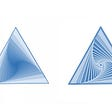## A Beginner’s Guide to Fibonacci Retracement Levels## Primes and The Powers of Two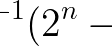## How do I predict the timing of Job using Astrology?## How to become a good problem solver?## Fibonacci Retracement: Why It’s The Best Tool In A Trader’s Kit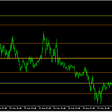## Puzzles in Quantum computing## Apoorva Panidapu

17 y/o math student, artist, and advocate for youth & gender minorities in STEAM. Winner of Strogatz Prize for Math Communication & Davidson Fellows Laureate.

## The Simplest Math Problem We Still Can’t Solve — Part 3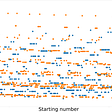## Bijective Proofs and Catalan Numbers## Applications of the Fibonacci Sequence## How to Argue Mathematically